Open in App
Not now

# Class 8 RD Sharma Solutions – Chapter 18 Practical Geometry – Exercise 18.5

• Last Updated : 06 May, 2021

### Question 1: Construct a quadrilateral ABCD given that AB = 4 cm, BC = 3 cm, ∠A = 75°, ∠B = 80° and ∠C = 120°?

Solution:

We have, AB = 4 cm, BC = 3 cm, ∠A = 75°, ∠B = 80° and ∠C = 120°.

Step 1: Draw a line segment AB = 4cm

Step 2: Construct an angle of 75° at A.

Step 3: Construct an angle of 80° at B.

Step 4: Taking B as the centre, draw an arc radius of 3 cm. Mark this point as C.

Step 5: Construct an angle of 120° at C such that it intersects the line segment AX. Mark that point as D.

Step 6: Now join BC, CD, and DA to form a quadrilateral.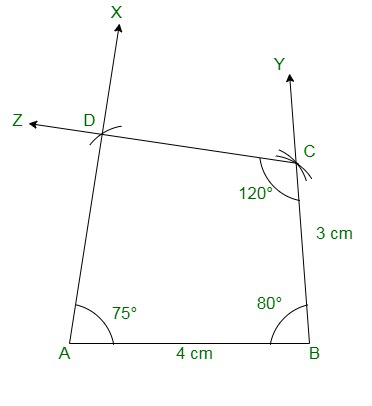### Question 2: Construct a quadrilateral ABCD where AB = 5.5 cm, BC = 3.7 cm, ∠A = 60°, ∠B = 105° and ∠D = 90°?

Solution:

We have, AB = 5.5 cm, BC = 3.7 cm, ∠A = 60°, ∠B = 105° and ∠D = 90°.

We know that ∠A + ∠B + ∠C + ∠D = 360°.

∴ ∠C = 105°

Step 1: Draw a line segment AB = 5.5cm

Step 2: Construct an angle of 60° at A.

Step 3: Construct an angle of 105° at B.

Step 4: Taking B as the centre, draw an arc radius of 3.7 cm. Mark this point as C.

Step 5: Construct and angle of 105° at C such that it intersects the line segment AX. Mark that point as D.

Step 6: Now join BC, CD, and DA to form a quadrilateral.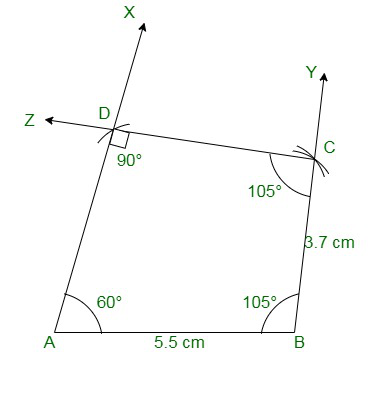### Question 3: Construct a quadrilateral PQRS where PQ = 3.5 cm, QR = 6.5 cm, ∠P = ∠R = 105° and ∠S = 75°?

Solution:

We have, PQ = 3.5 cm, QR = 6.5 cm, ∠P = ∠R = 105° and ∠S = 75°.

We know that ∠P + ∠Q + ∠R + ∠S = 360°

∴ ∠Q = 75°

Step 1: Draw a line segment PQ = 3.5cm

Step 2: Construct an angle of 105° at P.

Step 3: Construct an angle of 75° at Q.

Step 4: Taking Q as the centre, draw an arc radius of 6.5 cm. Mark this point as R.

Step 5: Construct an angle of 105° at R such that it intersects the line segment PX. Mark the point as S.

Step 6: Now join QR, RS, and PS to form a quadrilateral.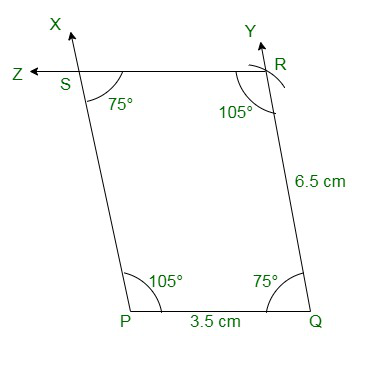### Question 4: Construct a quadrilateral ABCD when BC = 5.5 cm, CD = 4.1 cm, ∠A = 70°, ∠B = 110° and ∠D = 85°?

Solution:

We have, BC = 5.5 cm, CD = 4.1 cm, ∠A = 70°, ∠B = 110° and ∠D = 85°.

We know that ∠A + ∠B + ∠C + ∠D = 360°

∴ ∠C = 95°

Step 1: Draw a line segment BC = 5.5cm

Step 2: Construct an angle of 110° at B.

Step 3: Construct an angle of 95° at C.

Step 4: Taking C as the centre, draw an arc radius of 4.1 cm. Mark this point as D.

Step 5: Construct an angle of 85° at D such that it intersects the line segment BX. Mark that point as A.

Step 6: Now join CD, DA, and BA to form a quadrilateral.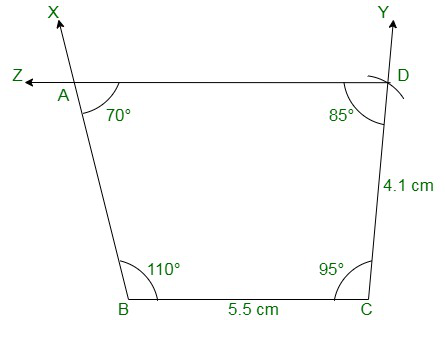### Question 5: Construct a quadrilateral ABCD ∠A = 65°, ∠B = 105°, ∠C = 75°, BC = 5.7 cm and CD = 6.8 cm?

Solution:

We have, ∠A = 65°, ∠B = 105°, ∠C = 75°, BC = 5.7 cm and CD = 6.8 cm.

We know that ∠A + ∠B + ∠C + ∠D = 360°

∴ ∠D = 115°

Step 1: Draw a line segment BC = 5.7cm

Step 2: Construct an angle of 105° at B.

Step 3: Construct an angle of 75° at C.

Step 4: Taking C as the centre, draw an arc radius of 6.8 cm. Mark this point as D.

Step 5: Construct an angle of 115° at D such that it intersects the line segment BX. Mark that point as A.

Step 6: Now join CD, DA, and BA to form a quadrilateral.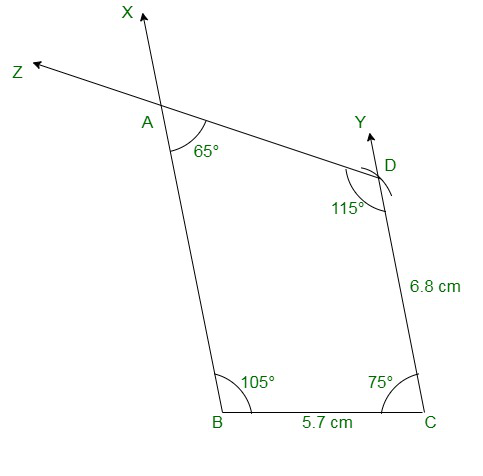### Question 6: Construct a quadrilateral PQRS in which PQ = 4 cm, QR = 5 cm ∠P = 50°, ∠Q = 110° and ∠R = 70°?

Solution:

We have, PQ = 4 cm, QR = 5 cm ∠P = 50°, ∠Q = 110° and ∠R = 70°.

Step 1: Draw a line segment PQ = 4cm

Step 2: Construct an angle of 50° at P.

Step 3: Construct an angle of 110° at Q.

Step 4: Taking Q as the centre, draw an arc radius of 5 cm. Mark this point as R.

Step 5: Construct an angle of 70° at R such that it intersects the line segment PX. Mark that point as S.

Step 6: Now join QR, RS, and PS to form a quadrilateral.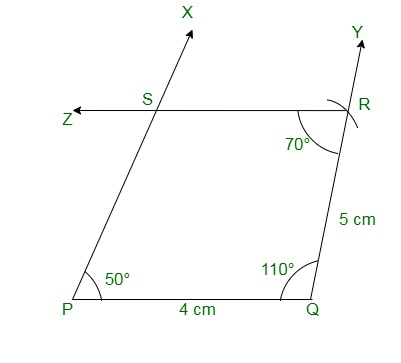My Personal Notes arrow_drop_up
Related Articles